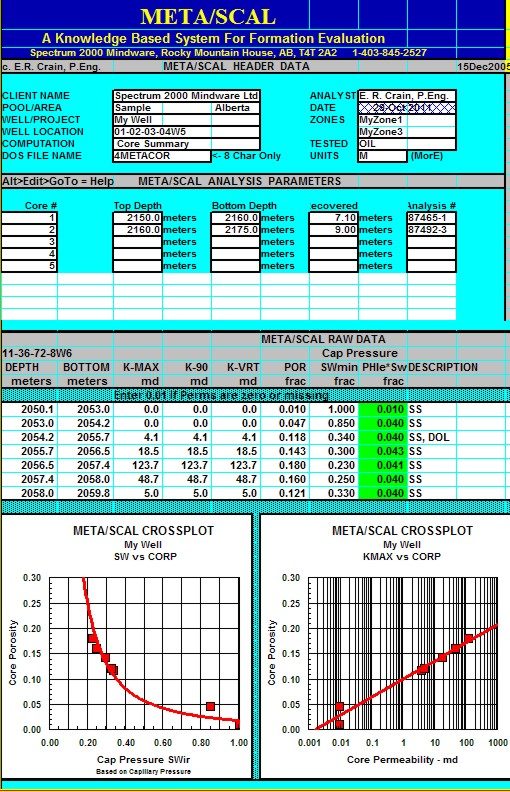Capillary pressuRE BASICS
Capillary pressure is a measurement of the force that draws a liquid up a thin tube, or capillary. Fluid saturation varies with the capillary pressure, which in turn varies with the vertical height above the free water level. Typically, laboratory measurements of capillary pressure are plotted on linear X - Y coordinate graph paper, as shown at the right.

Capillary pressure measured in the laboratory can be performed using air-brine or mercury injection (MICP) methods. The later is usually used in poorer quality reservoirs. The pressures involved are quite different so the graphs from the two methods are difficult to compare directly. By converting the pressure axis to height above free water (described later on this page), comparisons can be made quite easily.

Petrophysicists use cap pressure water saturation, adjusted for height above the free water level, and residual oil saturation (Sor) to help calibrate log derived water saturation in oil and gas reservoirs above the transition zone, and to help detect depleted reservoirs. It will not help calibrate SW in partially depleted zones.

The water saturations from capillary pressure measurements should be considered as "what-if" values. The saturations represent the value to expect IF the zone is hydrocarbon bearing. Clearly a water zone is still 100% wet, regardless of what the cap pressure SW appears to be.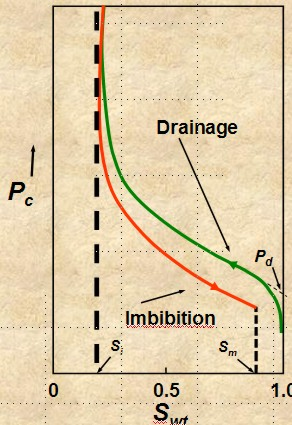drainage and imbibition
Accumulation of hydrocarbon in a reservoir is a drainage process and production by aquifer drive or waterflood is an imbibition process. The capillary pressure curve is different for these two processes, as shown in the illustration at the right. Most capillary pressure graphs show only the drainage curve.DRAINAGE
Fluid flow process in which the saturation of the nonwetting phase increases. Mobility of nonwetting fluid phase increases as nonwetting phase saturation increases - upper curve on image at right.IMBIBITION
Fluid flow process in which the saturation of the wetting
phase increases. Mobility of wetting phase increases
as wetting phase saturation increases - lower curve on image at right.THE PHYSICS OF CAPILLARY pressuRE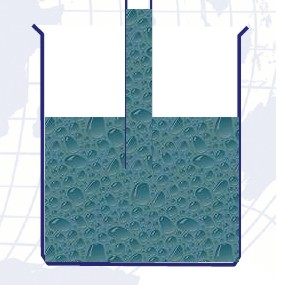If a glass capillary tube is placed in a large open vessel containing water, the combination of surface tension and wettability of tube to water will cause water to rise in the tube above the water level in the container outside the tube. The water will rise in the tube until the total force acting to pull the liquid upward is balanced by the weight of the column of liquid being supported in the tube. Assuming the radius of the capillary tube is R, the total upward force Fup, which holds the liquid up, is equal to the force per unit length of surface times the total length of surface:
1: Fup = 2 * PI * R * SIGgw * cos (THETA)

The upward force is counteracted by the weight of the water, which is a downward force equal to mass times acceleration:
2: Fdown = PI * R^2 * H * (DENSwtr - DENSair) * G

Assume density of air is negligible and set Fup = Fdown, solve for surface tension:
3: SIGgw = (R * H * DENSwtr * G) / (2 * cos (THETA))

In the more general case of oil and water, the equation becomes:
4: SIGow = (R * H * (DENSwtr - DENSoil)  * G) / (2 * cos(THETA))

Where:
PI = 3.1416.....
SIGgw = = surface tension between air and water (dynes/cm)
THETA =  contact angle
H = height of water in capillary (cm)
DENSwtr = density of water (gm/cc)
DENSair = density of air (gm/cc)
G = acceleration of gravity = 980.7 (cm/sec^2)

Rearranging equation 5:
5: H = (SIGgw * 2 * cos (THETA)) / (R * DENSwtr * G)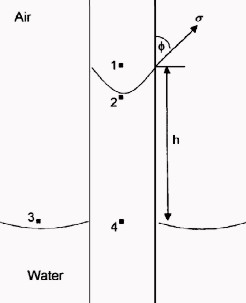The pressure difference across the interface between Points 1 and 2 is the capillary pressure:
6: P2 = P4 – G * H * DENSwtr
7: P1 = P3 – G * H * DENSair
8: Pc = P1 – P2

The pressure at Point 4 within the capillary tube is the same as that at Point 3 outside the tube.
9: Pc = (P3 – G * H * DENSair) – (P4 – G * H * DENSwtr)
10: Pc = G * H * (DENSwtr – DENSair)
11: Pc = G * H * ΔDENS

Where:
Pc = capillary pressure (dynes/cm2)
ΔDENS = density difference between the wetting and nonwetting
phase.(gn/cc)

Combining equations 5 and 11:
12: Pc = (SIGgw * 2 * cos (THETA)) / R

To convert Pc in dynes/cm2 to psi, multiply by 1.45 * 10^-5.

Thus if Pc is measured in the lab and SIGMA is known, equation 12 can be rearranged to give pore throat radius R.
13: R = (SIGgw * 2 * cos (THETA)) / Pc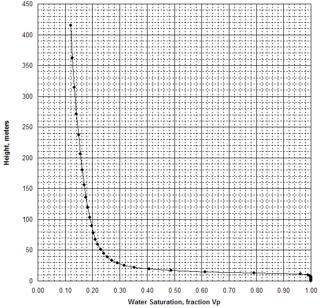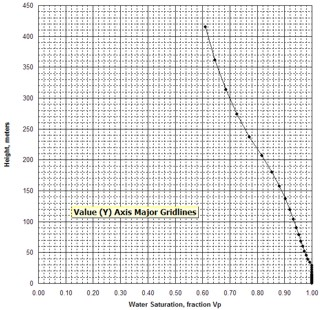Examples of capillary pressure curves in good quality rock (sample 1 – left) and poorer quality rock
(sample 2 – right)RESERVOIR QUALITY INDICATOR - LAMDA
There are four key parameters that are related to a capillary curve:

Si = irreducible wetting phase saturation

Sm = 1 - residual non-wetting phase saturation

Pd = displacement pressure, the pressure required to force non-wetting fluid into largest pores

LAMBDA = pore size distribution index; determines shape of capillary pressure curve

Si is the initial, or irreducible, water saturation in a hydrocarbon-bearing, water-wet, reservoir at initial pressure, prior to the start of production. It is termed SWir elsewhere in this Handbook.

(1 - Sm) is the residual oil saturation in a fully depleted water-wet reservoir, called Sor elsewhere in this Handbook.

(1 - SI) or (1 - SWir) is the non-wetting phase saturation at initial conditions prior to production. In a water-wet reservoir with a water drive, water saturation (Sw) increases above Si as production proceeds. At any time, saturation of the non-wetting phase (So) equals (1 - Sw).  In a gas expansion drive reservoir Sw = (1 - So) stays relatively constant over time. Capillary pressure curves help to explain the behaviour of water drive reservoirs but do little for gas expansion or gas cap reservoirs with no water drive.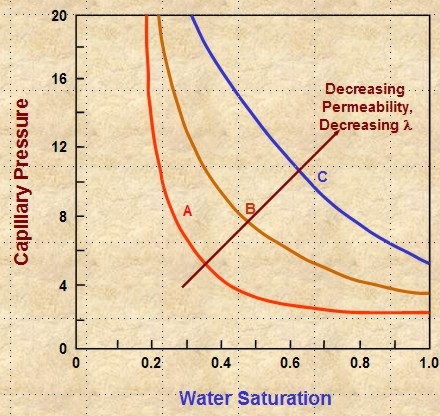A capillary pressure curve on Cartesian coordinates is difficult to fit with simple equations. By transforming the SW axis to Sw* and plotting Pc vs Sw* on log - log graph paper, the curves become straight lines.
14: Sw* = (Sw - SWir) / (1 - SWir - Sor)
15: Pc = Pd * (Sw*) ^ (1 / LAMBDA)

The slope of the line on the log-log graph is (-1 / LAMBDA) and the intercept at 100% Sw* is Pd. See illustration below. SWir is obtained from the Cartesian plot or on a saturation height graph (see next section on this web page).

Steeper slope equals higher (1 / LAMBDA), which means LAMBDA is lower. Lower LAMBDA means poorer quality reseryoir rock.

LAMBDA decreases with decreasing permeability, poor grain sorting, smaller grain size, and usually with lower porosity. These effects shift the cap pressure curve upward and to the right on Cartesian coordinate graphs, resulting in higher SWir values (see graph at the right).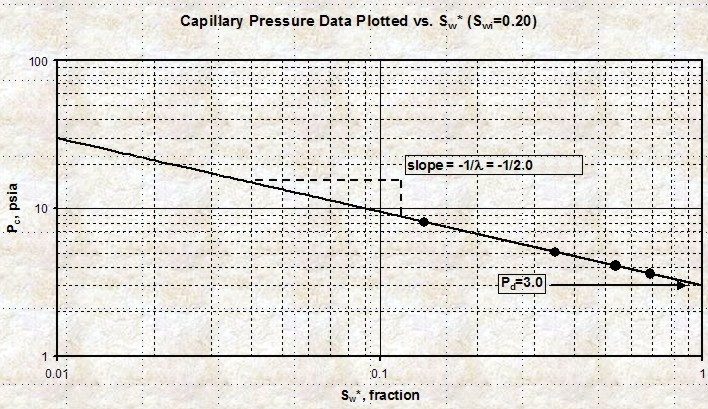Plot of Pc versus Sw* on log - log scale. The slope of the line is (-1 / LAMBDA) and the intercept
at 100% Sw* is Pd.

The typical range of (1 / LAMDA) is 0.5 for good quality sands to 4 or 8 for poor quality sands and carbonates. Type curve matching of Sw* can be used to assess cap pressure curves and reservoir quality.SATURATION - HEIGHT CURVES
To convert from laboratory measurements to reservoir conditions, we need to use the following relationship:
16: Pc_res = Pc_lab * (SIGow * cos (THETAow)) / (SIGgw * cos (THETAgw))Typical values for air-brine conversion to oil-water are:
SIGow = 24 dynes/cm
THETAow = 30 deg
SIGgw = 72 dynes/cm
THETAgw = 0 deg

Giving: Pc_res = 0.289 * Pc_lab

Solving equation 11 for H, and using reservoir (oil-water) Pc values:
17: H = KP15 * Pc_res / ΔDENS

Where:
Pc_res = capillary pressure at
reservoir (psi or KPa)
H = capillary rise (ft or meters)
ΔDENS = density difference (gm/cc)
KP15 = 2.308 (English units)
KP15 = 0.1064 (Metric units)

Tables and illustrations shown below in this Section were prepared by Dorian Holgate of Aptian Technical Ltd.

 Sw % Pc_lab Pc_res H 100 2 0.578 6.9 90 3 0.867 10.4 80 4 1.16 13.9 70 5 1.45 17.4 60 6 1.73 20.8 50 7 2.02 24.2 45 8 2.31 27.7 40 10 2.89 35 35 27 7.8 94 30 75 21.7 260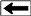Example of conversion of lab air-brine capillary pressure data to reservoir conditions, then into saturation-height H; results plotted in graph above.

All of the above assumes the lab data is an air-brine measurement. For mercury injection capillary, pressure (MICP) measurements, the density of the non-wetting phase (mercury) is 13.5 g/cc, so ΔDENS is much larger than the air-water case. As a result, Pc values from an MICP measurement are about 13.5 times higher than an air brine measurement (for the same SW value in the same core plug). To compare an air-brine cap pressure curve to an MICP curve, it is merely necessary to change the Pc scale on one of the graphs by the appropriate factor, or to convert both Pc scales to a saturation-height scale.

When H is calculated at a number of points on the Pc curve, the resulting graph of H vs SW is known as a saturation-height curve and can be plotted on a depth plot of log data or results by setting H = 0 at the base of transition zone on the logs. This assumes a uniform porosity-permeability regime, which is seldom encountered in real life, so more complicated methods are needed to superimpose the saturation values from multiple Pc curves.

If cap pressure curves are available at various depths in the reservoir, the pressure axis of each curve is converted to height above free water. Then the saturation from each curve is selected from the graph with respect to the sample's position above the water contact. These saturations are then plotted with respect to the sample depths onto the log analysis depth plot, as shown in the example below.

The example below was prepared by Dorian Holgate during one of our joint projects.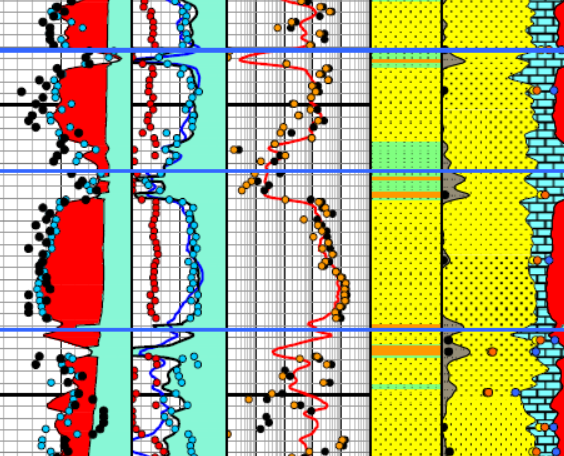Enlarged image of log analysis depth plot showing porosity, saturation, permeability, a

Enlarged image of log analysis depth plot showing porosity, saturation, permeability, and lithology tracks over a conventional oil-bearing sandstone. Black dots are conventional core porosity and permeability. Pink dots show porosity of samples used for cap pressure measurements and the water saturation for those samples, chosen from their respective height above free water  curve. The pink dots match the log analysis water saturation (blue curve) very closely everywhere.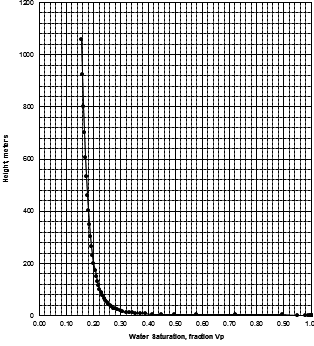MICP capillary pressure curve (left) and equivalent height above free water version (right) for the sample just above the oil water contact on the above example. The reservoir is only 30 meters thick, so we are only interested in a very small portion of these graphs, near the bottom of each. The graph has no resolution at low height values so it is easier to use the equivalent table of values, or re-plot the data on a more appropriate scale.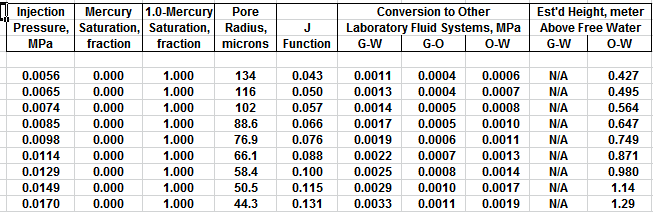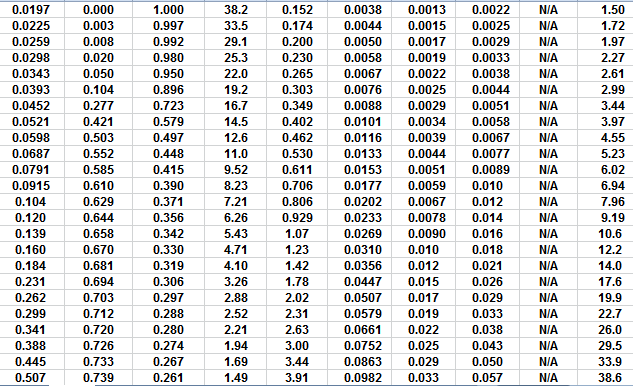The first Pc sample above the oil-water contact is at a height of 4.5 meters above the contact. The nearest height in the table is 4.55 meters (column 10) and the corresponding saturation (column 3) is 0.497. Use interpolation or plot a detailed graph for better accuracy. Repeat this for each sample and its respective data table.FINDING IRREDUCIBLE WATER SATURATION
It has been traditional to look at the minimum water saturation on a cap pressure curve and to call it irreducible water saturation (SWir). In the above example, we don't see the minimum until 600 to 800 meters above the oil -water contact, and this reservoir is only 30 meters thick. The true irreducible water saturation is much higher than the minimum on the graph because we are so close to the contact.

The true irreducible saturation is defined by the height versus SW curve for each sample, and not by the minimum SW. If porosity, permeability, pore geometry, grain size, sorting vary in a reservoir, you need a height versus SW curve for each rock type, and a reliable method for identifying those rock types by using a log analysis algorithm or curve shape pattern.

Tables and illustrations shown below in this Section were prepared by Dorian Holgate of Aptian Technical Ltd.

A typical method to differentiate rock types is to use raw log or analysis results ranges to segregate rocks into various categories. Then an appropriate cap pressure curve  can be applied to each rock type, where ever it occurs in the reservoir interval. An example is shown in the table below: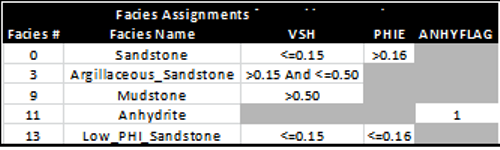Example of facies assignments using cutoff ranges based on analysis results. Note that only
facies 0, 3, and 13 are reservoir facies.

Once facies are assigned, the cap pressure curves are segregated according to facies and converted to height above free water level (HAFWL):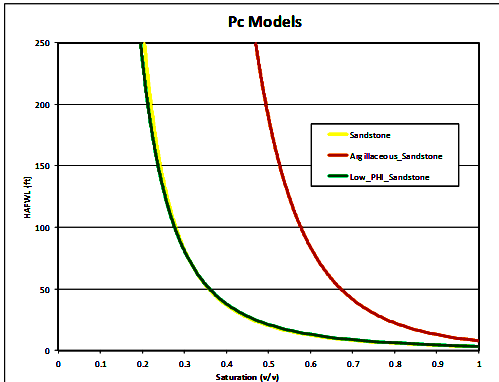Height above free water level (HAFWL) curves for the 3 reservoir facies. Note that the yellow (facies 0) and
green (facies 13) curves are virtually identical, so there are in fact only two rock types.

The equation used to model water saturation from capillary pressure is:
18: SWcp = A * ((Perm / PHIe)^0.5 * HAFWL)^B + C

Where:
Perm = permeability (mD)
PHIe = effective porosity (fractional)
HAFWL = height above free water level (meters)
A, B, C = regression coefficients

The coefficients can be obtained with Excel Solver or commercial Pc software.

For the cap pressure curves shown above, the coefficients A, B, and C are: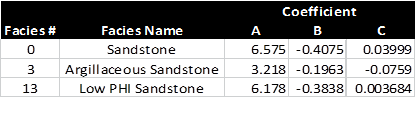Regression coefficients for 3 cap pressure curves. Note that results for facies 0 and 13 are virtually identical.

A comparison of the capillary pressure derived water saturation and that from a conventional Simandoux porosity-resistivity model is shown below.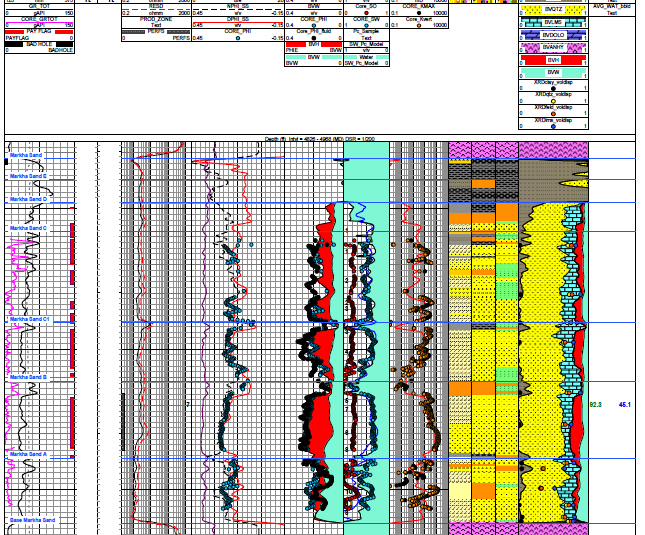Example showing SWpc (black), SWsimandoux (blue), SWcore (black dots), and SOcore (red dots) in Track 5. Note the near perfect match between log, core, and cap pressure saturations. Log and core porosity are in Track 4 and permeability is in Track 6. Unless porosity and permeability match core, it will be impossible to match the saturations.MEASURING Capillary pressure
Capillary pressure can be measured in the laboratory in four different ways:

Porous diaphragm method
•   Mercury injection method
Centrifuge method
Dynamic method

Detailed operation of the laboratory equipment is beyond the scope of this Handbook. The illustrations are not fully self-explanatory, but the general principles are relatively visible.POROUS DIAPHRAGM METHOD
The apparatus and sample data set are shown below. The method is very accurate but it can take days to months to get a complete cap pressure curve.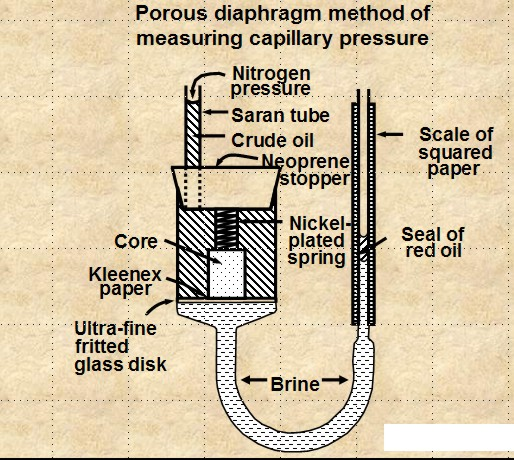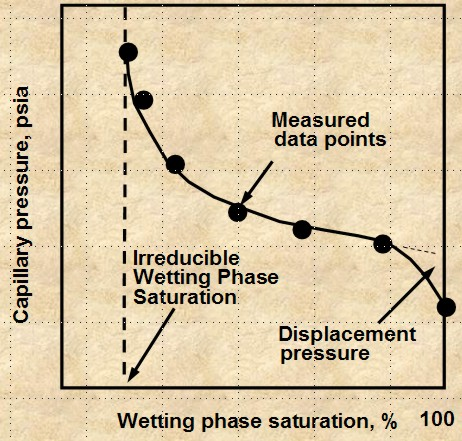Porous Diaphragm apparatus and results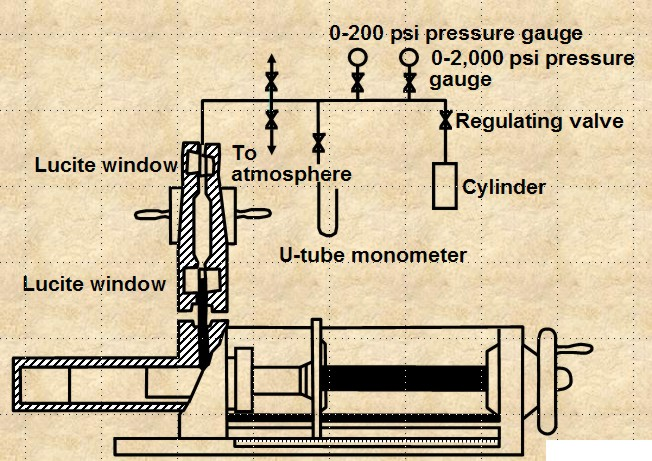MERCURY INJECTION METHOD
The apparatus is shown at right. The method is reasonably accurate and takes minutes to hours to get a complete cap pressure curve. The core sample cannot be re-used for any purpose and require special disposal procedures due to the mercury. A conversion factor is needed to get an equivalent air-brine capillary pressure, comparable to that from a porous plate method.CENTRIFUGAL METHOD
The apparatus is shown below. The method is reasonably accurate and takes hours to days to get a complete cap pressure curve. Data analysis is complicated and can lead to errors.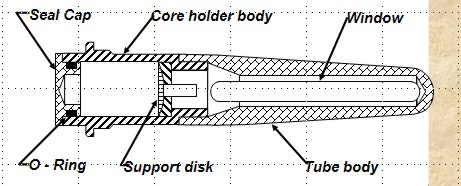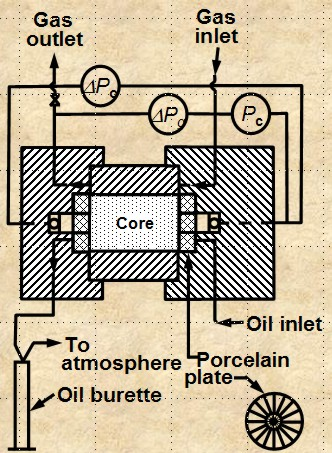DYNAMIC METHOD
The apparatus is shown at right. The method is reasonably accurate and simulates actual reservoir flow when whole core is used. It can take weeks or months to complete a full cap pressure curve.AVERAGING Capillary pressure
-- Leverett J-FUNCTION
The Leverett J-function was originally an attempt to convert all capillary pressure data to a universal curve. A universal capillary pressure curve does not exist because the rock properties affecting capillary pressures in reservoir have extreme variation with lithology (rock type). But, Leverett’s J-function has proven valuable for correlating capillary pressure data within a lithologic rock type.

The Leverett J-Function is described by
21: Jsw =  C * Pc * ((K / PHIe)^0.5) / (SIGwo * Cos (THETA))

The square root of (K / PHIe) is a function of pore throat radius. The constant C is a units conversion factor to make Jsw unitless.

By substitution and rearrangement:
22: H = Jsw * SIGwo * cos (THETA) / ((K / PHIe)^0.5) / ΔDENS

All parameters are adjusted to represent reservoir conditions. When Pc is in psi and ΔDENS is in psi/ft, then H is in feet.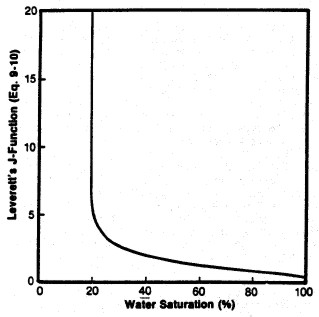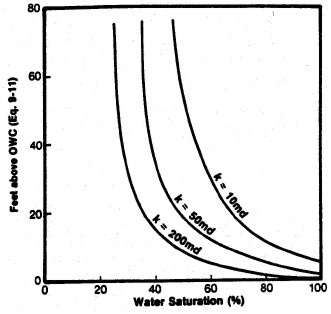Left: Smoothed Jsw curve versus Sw; Right: Derived saturation-height curves for various permeability values (porosity held constant) using equation 22.

J-function is useful for averaging capillary pressure data from a given rock type from a given reservoir and
can sometimes be extended to different reservoirs having the same lithology. Use extreme caution in assuming this can be done. J-function is usually not an accurate correlation for different lithologies. If J-functions are not successful in reducing the scatter in a given set of data, then this suggests that we are dealing with variation in rock type.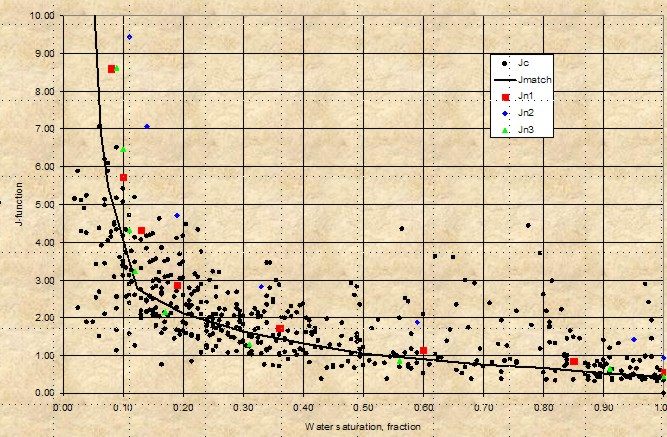Leverett J-Function versus water saturation for West Texas Carbonates showing moderate spread of saturation data - rock type is not really very uniform (varying pore geometry). However, the J-function curve may be suitable for reservoir simulation purposesCAP PRESSURE EXAMPLE - Sand and Silt Reservoir
A capillary pressure (Pc) data set, along with some calculated parameters, is summarized in the table below.

 CAPILLARY PRESSURE SUMMARY Sample Depth Perm PHIe SWir SWir PHI*SW PHI*SW sqrt/PHIe) Pore Throat m mD 425m 100m 425m 100m Radius um Bakken 1 x03.5 2.40 0.118 0.12 0.19 0.014 0.022 4.51 1.358 2 x04.3 0.24 0.137 0.62 0.94 0.085 0.129 1.32 0.036 3 x04.5 0.32 0.139 0.39 0.64 0.054 0.089 1.52 0.100 4 x05.2 0.77 0.149 0.31 0.62 0.046 0.092 2.27 0.113 Average x04.4 0.93 0.136 0.36 0.60 0.050 0.083 2.41 0.402 Torquay 5 x16.8 0.05 0.163 1.00 1.00 0.163 0.163 0.55 0.008 6 x20.4 0.07 0.145 0.59 0.97 0.086 0.141 0.69 0.038 7 x21.8 0.09 0.174 0.79 0.96 0.137 0.167 0.72 0.019 8 x23.8 0.03 0.157 1.00 1.00 0.157 0.157 0.44 0.009 9 x31.4 0.07 0.138 0.83 0.98 0.115 0.135 0.71 0.017 Average x24.4 0.07 0.154 0.80 0.98 0.124 0.150 0.64 0.021In higher permeability rock, the cap pressure curve quickly reaches an asymptote and the minimum saturation usually represents the actual water saturation in an undepleted hydrocarbon reservoir above the transition zone. In tight rock, the asymptote is seldom reached, so we pick saturation values from the cap pressure curves at two heights (or equivalent) Pc values to represent two extremes of  reservoir condition.

Only sample 1 in the above table behaves close to asymptotically, as in curve A in the schematic illustration at the right. All other samples behave like curves B and C (or worse). The real cap pressure curves for samples 1 and 2 are shown below.Examples of capillary pressure curves in good quality rock (sample 1 – left) and poorer quality rock
(sample 2 – right)

The summary table shows wetting phase saturation selected by observation of  the cap pressure graphs at two different heights above free water, namely 100 meters and 425 meters in this example. In this case, the 100 meter data gives water saturations that we commonly see in petrophysical analysis of well logs in hydrocarbon bearing Bakken reservoirs in Saskatchewan. This is a pragmatic way to indicate the water saturation to be expected when a Bakken reservoir is at or near irreducible water saturation. The data for the 450 meter case is considerably lower and probably does not represent reservoir conditions in this region of the Williston Basin.

Two other columns in the table are calculated from the primary measurements.

The first is the product of porosity times saturation, PHI*SW, often called Buckle’s Number. It is considered to be a measure of pore geometry or grain size. Higher values are finer grained rocks. These values vary considerably in the Bakken, between low and medium values, indicating the laminated nature of the silt / sand reservoir. The values in the Torquay are uniformly high, indicating that the reservoir is poor quality in all samples.

The second is the square root of permeability divided by porosity, sqrt(Kmax/PHIe), which is another measure of reservoir quality, directly proportional to pore throat radius and Pc. High numbers represent good connectivity and low values show poor connectivity. Again, the Bakken shows the variations due to laminations, and the Torquay shows low values and unattractive reservoir quality.

Average pore throat radius and detailed pore throat distribution data are now routinely available in the capillary pressure spreadsheet provided by the core analysis laboratory. Examples are shown below.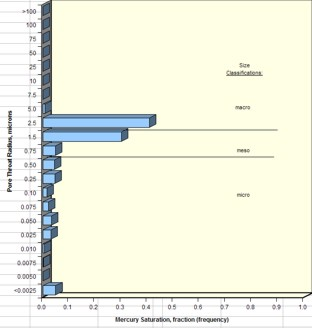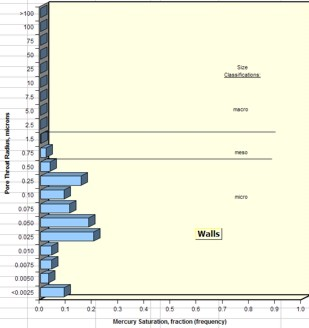Examples of pore throat radius distribution in good quality rock (sample 1 – left) and poorer quality rock (sample 2 – right)

By comparing cap pressure and pore throat distribution graphs from each sample with the quality indicator values in the summary table, it becomes more evident as to which parameters in a petrophysical analysis might be the best indicator of reservoir quality. Since both Buckle’s Number and the Kmax/PHIe parameter can be determined from logs, it has been relatively common to assess reservoir quality from these parameters as a proxy for capillary pressure and pore throat measurements.

However, in thinly laminated reservoirs like the Bakken, this is not always possible since the logging tools average 1 meter of rock. This means we cannot see the internal variations of rock quality evident in the core data."META/SCAL" Spreadsheet -- Capillary Pressure Summary
This spreadsheet is used to summarize Capillary Pressure data, with crossplot to find SWir.